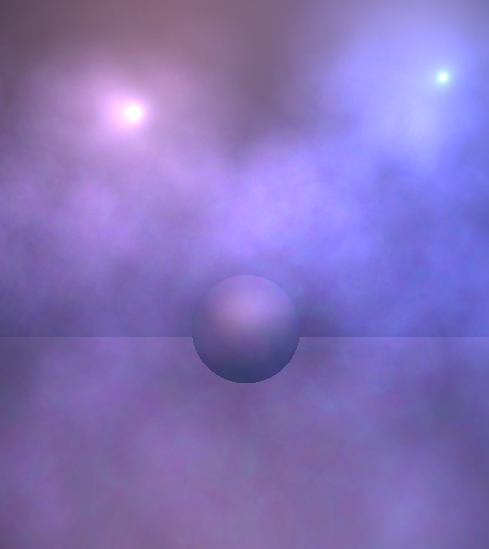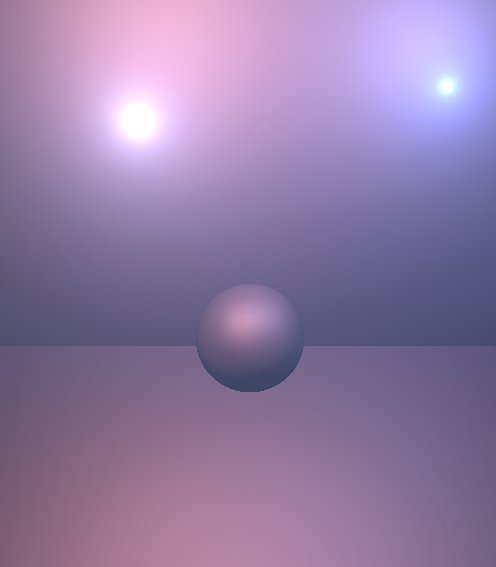## In-Scattering Demo

This demo shows an analytic solution to the differential in-scattering equation for light in participating media. It's a similar but simplified version of equations found in ,  and as I recently discovered . However I thought showing the derivation might be interesting for some out there, plus it was a good excuse for me to brush up on my $$\LaTeX$$.

You might notice I also updated the site's theme, unfortunately you need a white background to make wordpress.com LaTeX rendering play nice with RSS feeds (other than that it's very convenient).

The demo uses GLSL and shows point and spot lights in a basic scene with some tweakable parameters:### Background

Given a view ray defined as:

$\mathbf{x}(t) = \mathbf{p} + t\mathbf{d}$

We would like to know the total amount of light scattered towards the viewer (in-scattered) due to a point light source. For the purposes of this post I will only consider single scattering within isotropic media.

The differential equation that describes the change in radiance due to light scattered into the view direction inside a differential volume is given in PBRT (p578), if we assume equal scattering in all directions we can write it as:

$dL_{s}(t) = \sigma_{s}L_{i}(t)\,dt$

Where $$\sigma_{s}$$  is the scattering probability which I will assume includes the normalization term for an isotropic phase funtion of $$\frac{1}{4pi}$$. For a point light source at distance d with intensity I we can calculate the radiant intensity at a receiving point as:

$L_{i} = \frac{I}{d^2}$

Plugging in the equation for a point along the view ray we have:

$L_{i}(t) = \frac{I}{|\mathbf{x}(t)-\mathbf{s}|^2}$

Where s is the light source position. The solution to (1) is then given by:

$L_{s} = \int_{0}^{d} \sigma_{s}L_{i}(t) \, dt$

$L_{s} = \int_{0}^{d} \frac{\sigma_{s}I}{|\mathbf{x}(t)-\mathbf{s}|^2}\,dt$

To find this integral in closed form we need to expand the distance calculation in the denominator into something we can deal with more easily:

$L_{s} = \sigma_{s}I\int_0^d{\frac{dt}{(\mathbf{p} + t\mathbf{d} - \mathbf{s})\cdot(\mathbf{p} + t\mathbf{d} - \mathbf{s})}}$

Expanding the dot product and gathering terms, we have:

$L_{s} = \sigma_{s}I\int_{0}^{d}\frac{dt}{(\mathbf{d}\cdot\mathbf{d})t^2 + 2(\mathbf{m}\cdot\mathbf{d})t + \mathbf{m}\cdot\mathbf{m} }$

Where $$\mathbf{m} = (\mathbf{p}-\mathbf{s})$$.

Now we have something a bit more familiar, because the direction vector is unit length we can remove the coefficient from the quadratic term and we have:

$L_{s} = \sigma_{s}I\int_{0}^{d}\frac{dt}{t^2 + 2bt + c}$

At this point you could look up the integral in standard tables but I'll continue to simplify it for completeness.  Completing the square we obtain:

$L_{s} = \sigma_{s}I\int_{0}^{d}\frac{dt}{ (t^2 + 2bt + b^2) + (c-b^2)}$

Making the substitution (u = (t + b)), (v = (c-b2){1/2}) and updating our limits of integration, we have:

$L_{s} = \sigma_{s}I\int_{b}^{b+d}\frac{du}{ u^2 + v^2}$

$L_{s} = \sigma_{s}I \left[ \frac{1}{v}\tan^{-1}\frac{u}{v} \right]_b^{b+d}$

Finally giving:

$L_{s} = \frac{\sigma_{s}I}{v}( \tan^{-1}\frac{d+b}{v} - \tan^{-1}\frac{b}{v} )$

This is what we will evaluate in the pixel shader, here's the GLSL snippet for the integral evaluation (direct translation of the equation above):

float InScatter(vec3 start, vec3 dir, vec3 lightPos, float d)
{
// light to ray origin
vec3 q = start - lightPos;

// coefficients
float b = dot(dir, q);
float c = dot(q, q);

// evaluate integral
float s = 1.0f / sqrt(c - b*b);
float l = s * (atan( (d + b) * s) - atan( b*s ));

return l;
}


Where d is the distance traveled, computed by finding the entry / exit points of the ray with the volume.

To make the effect more interesting it is possible to incorporate a particle system, I apply the same scattering shader to each particle and treat it as a thin slab to obtain an approximate depth, then simply multiply by a noise texture at the end.### Optimisations

• As it is above the code only supports lights with infinite extent, this implies drawing the entire frame for each light.  It would be possible to limit it to a volume but you'd want to add a falloff to the effect to avoid a sharp transition at the boundary.
• Performing the full evaluation per-pixel for the particles is probably unnecessary, doing it at a lower frequency, per-vertex or even per-particle would probably look acceptable.

• Generally objects appear to have wider specular highlights and more ambient lighting in the presence of particpating media.   Discusses this in detail but you can fudge it by lowering the specular power in your materials as the scattering coefficient increases.
• According to Rayliegh scattering blue light at the lower end of the spectrum is scattered considerably more than red light.  It's simple to account for this wavelength dependence by making the scattering coefficient a constant vector weighted towards the blue component.  I found this helps add to the realism of the effect.
• I'm curious to know how the torch light was done in Alan Wake as it seems to be high quality (not just billboards) with multiple light shafts.. maybe someone out there knows?